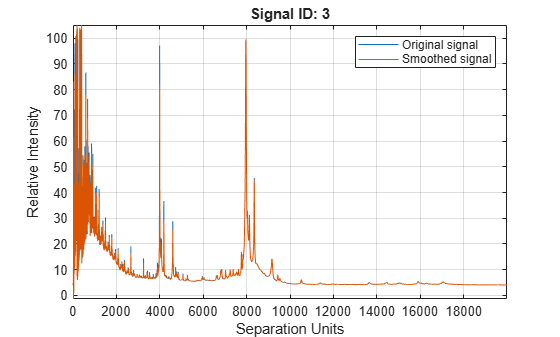Main Content

# mssgolay

Smooth signal with peaks using least-squares polynomial

## Syntax

```Yout = mssgolay(X, Intensities) mssgolay(X, Intensities, ...'Span', SpanValue, ...) mssgolay(X, Intensities, ...'Degree', DegreeValue, ...) mssgolay(X, Intensities, ...'ShowPlot', ShowPlotValue, ...) ```

## Arguments

 `X` Vector of separation-unit values for a set of signals with peaks. The number of elements in the vector equals the number of rows in the matrix `Intensities`. The separation unit can quantify wavelength, frequency, distance, time, or m/z depending on the instrument that generates the signal data. `Intensities` Matrix of intensity values for a set of peaks that share the same separation-unit range. Each row corresponds to a separation-unit value, and each column corresponds to either a set of signals with peaks or a retention time. The number of rows equals the number of elements in vector `X`.

## Description

Tip

Use the following syntaxes with data from any separation technique that produces signal data, such as spectroscopy, NMR, electrophoresis, chromatography, or mass spectrometry.

`Yout = mssgolay(X, Intensities)` smooths raw noisy signal data, `Intensities`, using a least-squares digital polynomial filter (Savitzky and Golay filters). The default span or frame is `15` samples.

```mssgolay(X, Intensities, ...'PropertyName', PropertyValue, ...)``` calls `mssgolay` with optional properties that use property name/property value pairs. You can specify one or more properties in any order. Each `PropertyName` must be enclosed in single quotation marks and is case insensitive. These property name/property value pairs are as follows:

``` mssgolay(X, Intensities, ...'Span', SpanValue, ...)``` modifies the frame size for the smoothing function. If `SpanValue` is greater than `1`, the window is the size of `SpanValue` in samples independent of the `X` vector. Higher values smooth the signal more with an increase in computation time. If `SpanValue` is less than `1`, the window size is a fraction of the number of points in the input data, `X`. For example, if `SpanValue` is `0.05`, the window size is equal to `5%` of the number of points in `X`.

Note

The original algorithm by Savitzky and Golay assumes the input vector, `X`, has uniformly spaced separation units, while `mssgolay` also allows one that is not uniformly spaced. Therefore, the sliding frame for smoothing is centered using the closest samples in terms of the `X` value and not in terms of the `X` index.

When the input vector, `X`, does not have repeated values or NaN values, the algorithm is approximately twice as fast.

When the input vector, `X`, is evenly spaced, the least-squares fitting is performed once so that the signal is filtered with the same coefficients, and the speed of the algorithm increases considerably.

If the input vector, `X`, is evenly spaced and `SpanValue` is even, span is incremented by `1` to include both edge samples in the frame.

```mssgolay(X, Intensities, ...'Degree', DegreeValue, ...)``` specifies the degree of the polynomial (`DegreeValue`) fitted to the points in the moving frame. The default value is `2`. `DegreeValue` must be smaller than `SpanValue`.

```mssgolay(X, Intensities, ...'ShowPlot', ShowPlotValue, ...)``` plots smoothed signals over the original. When `mssgolay` is called without output arguments, the signals are plotted unless `ShowPlotValue` is `false`. When `ShowPlotValue` is `true`, only the first signal in `Intensities` is plotted. `ShowPlotValue` can also contain an index to one of the signals in `Intensities`.

## Examples

collapse all

This example shows how to smooth mass spectrometry data using least-squares polynomial approach.

Load a MAT-file, included with Bioinformatics Toolbox™, that contains mass spectrometry data including `MZ_lo_res` , a vector of m/z values for a set of spectra, and `Y_lo_res` , a matrix of intensity values for a set of mass spectra that share the same m/z charge.

`load sample_lo_res`

Apply least-squares polynomial smoothing to the data.

`YS = mssgolay(MZ_lo_res, Y_lo_res);`

Plot the third sample/spectrogram in `Y_lo_res` , and its smoothed signal.

`mssgolay(MZ_lo_res,Y_lo_res,'SHOWPLOT',3);`Introduced before R2006a

## Support평가판 신청